#### You may also like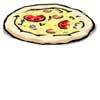### Pizza Portions

My friends and I love pizza. Can you help us share these pizzas equally?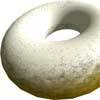### Doughnut

How can you cut a doughnut into 8 equal pieces with only three cuts of a knife?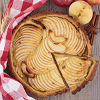### Pies

Grandma found her pie balanced on the scale with two weights and a quarter of a pie. So how heavy was each pie?

# A4 Fraction Addition

##### Age 7 to 11Challenge Level

Find a partner and start with a sheet of A4 paper and a pair of scissors each. Have a go at the activity below and compare what you've made!

The whole sheet of A4 paper represents 1 whole.

Take your sheet of paper and fold it in half into two rectangles. Unfold it and cut along the fold line. You might end up with two pieces which look like the two red rectangles below. Each of these rectangles represents $\frac{1}{2}$.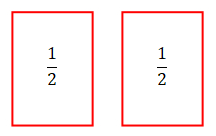Take one of your rectangles and cut it in half into two smaller rectangles in the same way. By halving this you now have two rectangles which each represent $\frac{1}{4}$, like the blue rectangles below: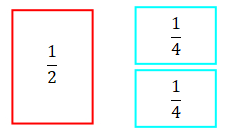Depending on how you chose to cut the rectangle in half, your $\frac{1}{4}$ rectangles might look different to this - they might be long and thin, like the rectangles below: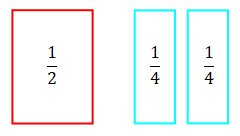Now, take one of your $\frac{1}{4}$ rectangles and cut it in half. You will then have two new rectangles which both represent $\frac{1}{8}$ like the green rectangles below.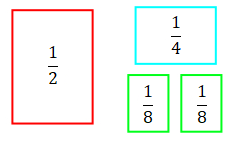Halve one of your green rectangles to get two $\frac{1}{16}$ rectangles like the orange one below. Then throw away one of these $\frac{1}{16}$ rectangles so that you have one rectangle of each size.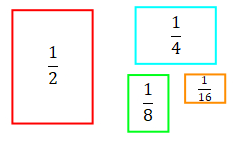At this point, stop and compare your rectangles with your partner's rectangles. Do they look the same? Are they different to the ones in the picture above?

Imagine that you made your $\frac{1}{2}$ rectangle the same as the red rectangle above, but then you cut all your other rectangles to be long and thin. Your rectangles might now look like this: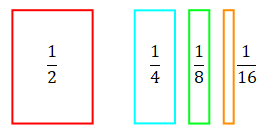Are there any different rectangles that we could have chosen?

Now, get four pieces of A4 paper and attach one to each of your fraction pieces. Remember that the full piece of A4 paper is counted as "one whole".

You should end up with combinations that look a bit like the ones below. These are mixed numbers of pieces of A4 paper now, because they have a whole number part and a fraction part.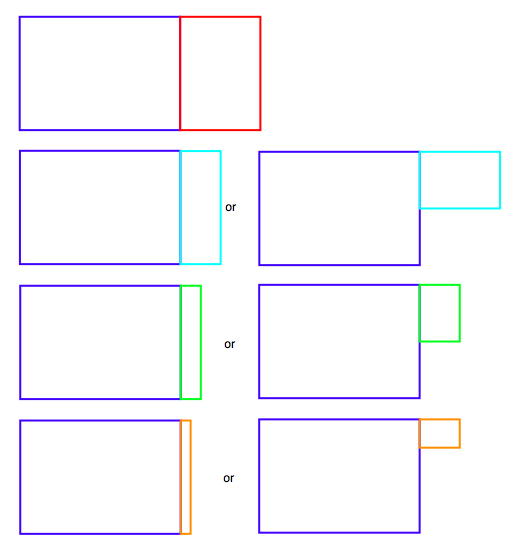Can you work out what mixed number is represented by each?

Take one of your mixed number shapes and put it with one that your partner has made. Write down the addition calculation and the answer.

For example, the addition below would give you 1$\frac{1}{4}$ + 1$\frac{1}{2}$ = 2$\frac{3}{4}$.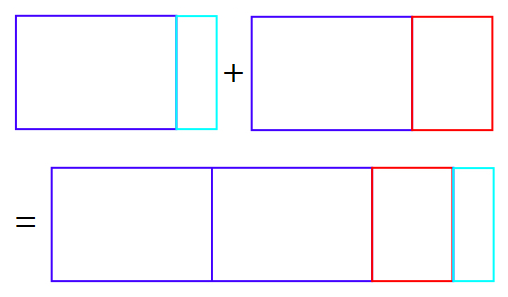How many different addition number sentences can you make?

You might also like to try a subtraction version of this task.